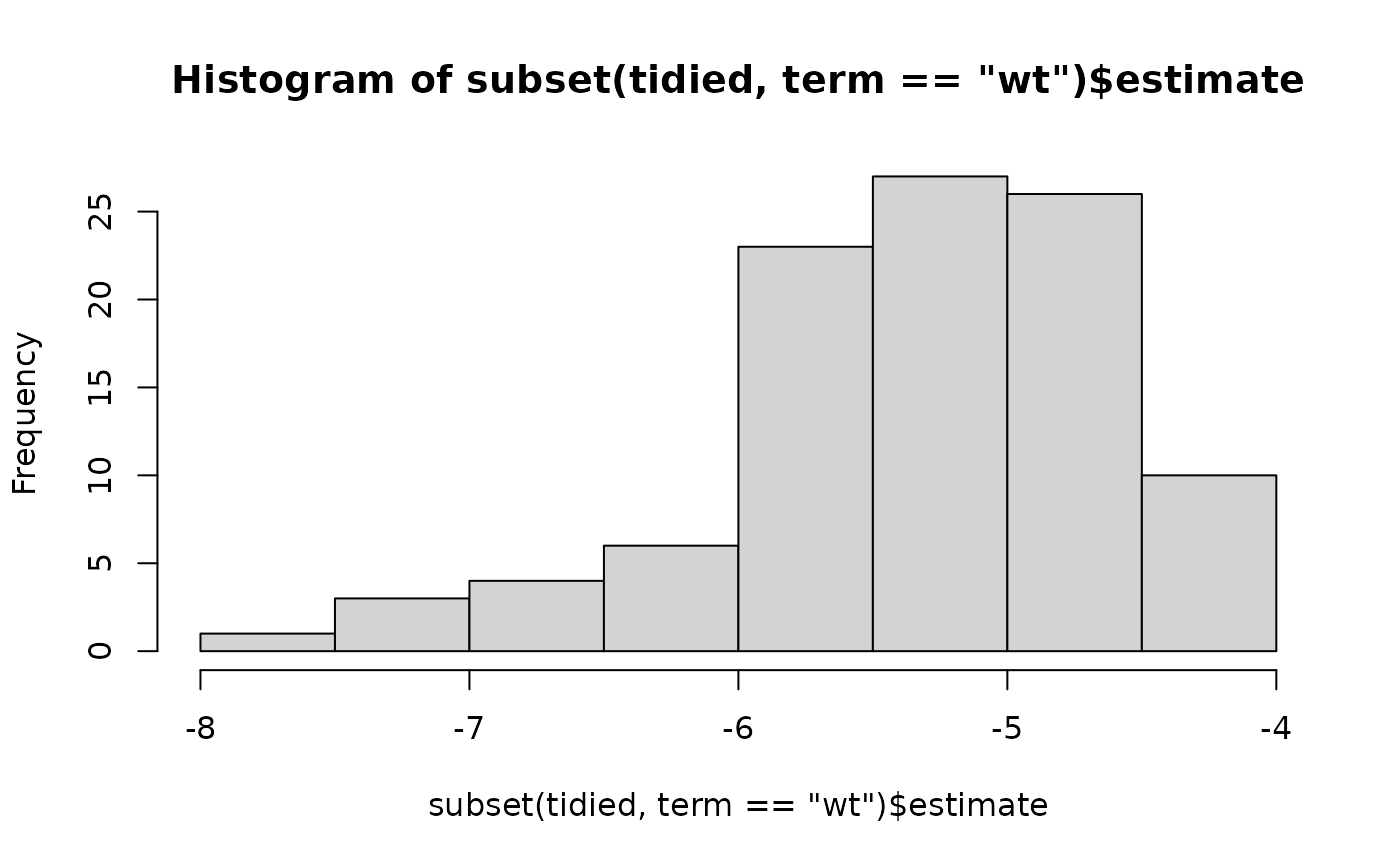Generate n bootstrap replicates.

bootstrap(data, n, id = ".id")

## Arguments

data A data frame Number of bootstrap replicates to generate Name of variable that gives each model a unique integer id.

## Value

A data frame with n rows and one column: strap

## See also

Other resampling techniques: resample_bootstrap(), resample_partition(), resample()

## Examples

library(purrr)
boot <- bootstrap(mtcars, 100)

models <- map(boot$strap, ~ lm(mpg ~ wt, data = .)) tidied <- map_df(models, broom::tidy, .id = "id") hist(subset(tidied, term == "wt")$estimate)hist(subset(tidied, term == "(Intercept)")\$estimate)